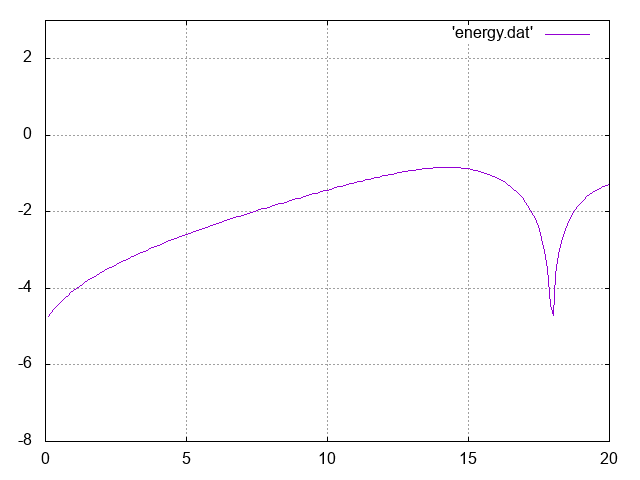## SelalibTags:

Modular library for kinetic and gyrokinetic simulations of plasmas in fusion energy devices by semi-Lagrangian or particle-in-cell methods.## UNIFORMLY ACCURATE PARTICLE-IN-CELL METHOD FOR HIGHLY OSCILLATORY VLASOV-POISSON EQUATION

### AllGO instructions

If you run the application in allgo be warn that the duration time could be very long if you use a lot of particles.

#### Inputs

You should upload one file for each job. A text data file:

You will find some instructions to create your data file below. There is an example in Demo.

#### Parameters

The filename parameter should be the name of your input file.

ie. for an input file called data.txt, the filename parameter should be : "data.txt"

#### General information

This simulation is the code for the paper

Uniformly accurate particle-in-cell method for the long time two-dimensional Vlasov-Poisson equation with strong magnetic field

by Nicolas Crouseilles, Mohammed Lemou, Florian Mehats and Xiaofei Zhao

This application is running the numerical simulation of a Vlasov-Poisson equation modeling charged particles in a beam submitted to a highly oscillatory external electric field. The numerical scheme is designed to be uniformly accurate with respect to the size of the fast time oscillations of the solution, which means that no time step refinement is required to simulate the problem.

The scheme combines the Particle-In-Cell method with a class of Uniformly Accurate (UA) time integrators to solve the characteristics. These UA time integrators are derived by means of a two-scale formulation of the characteristics, with the introduction of an additional periodic variable.

If you get the selalib software you can run the code in your build directory. Copy the data file:

``````\$ selalib/simulations/parallel/pic_vp_2d2v_cart_tau/data.txt
``````
• Run with the command
``````\$ mpirun -np 4 ./bin/sim_pic_vp_2d2v_cart_tau data.txt
``````

ntau value is the max for processors count.

You get the first fourier modes of energy in file energy.dat

You can run the numerical experiments changing the following parameters:

• `nstepmax` : maximum time steps.
• `nx` : size in x.
• `ny` : size in y.
• `tfinal` : finaltime.
• `ntau` : number of points for time discretization.
• `npm` : number of particles by cell.
• `ep` : epsilon.
• `alpha` : perturbation amplitude (named eta in the paper).
• `kx` : wave number in x (named k in the paper).
• `ky` : wave number in y.
• `dt` : time step.
• `plot` : (plot rho and df) (.true. or .false.)

The data file 'data.txt' must be a fortran namelist, here an example:

``````\$donnees
nstepmax = 10000
nx       = 64
ny       = 32
tfinal   = 25.
ntau     = 16
npm      = 100
ep       = 0.005
alpha    = 0.02
kx       = 0.5
ky       = 1.0
dt       = 0.1
plot     = .false.
\$end
``````In input :

data.txt
```\$donnees
nstepmax = 10000
nx       = 64
ny       = 32
tfinal   = 20.0
ntau     = 8
npm      = 10
ep       = 0.005
alpha    = 0.02
kx       = 0.5
ky       = 1.0
dt       = 0.1
plot     = .false.
\$end
```

In output :

selalib.png
``

20/09/2016 : Version 1.2, Fix diagnostics and add total energy and RMS
18/09/2016 : Version 1.1, Fix an error in boundary conditions of rho
16/09/2016 : Version 1.0, Improve the documentation

#### How to use our REST API :

Think to check your private token in your account first. You can find more detail in our documentation tab.

This app id is : 136

This curl command will create a job, and return your job url, and also the average execution time

files and/or dataset are optionnal, think to remove them if not wanted
```curl -H 'Authorization: Token token=<your_private_token>' -X POST
-F job[webapp_id]=136
-F job[param]=""
-F job[queue]=standard
-F files=@test.txt
-F files=@test2.csv
-F job[file_url]=<my_file_url>
-F job[dataset]=<my_dataset_name> https://allgo.inria.fr/api/v1/jobs
```

Then, check your job to get the url files with :

```curl -H 'Authorization: Token token=<your_private_token>' -X GET https://allgo.inria.fr/api/v1/jobs/<job_id>
```